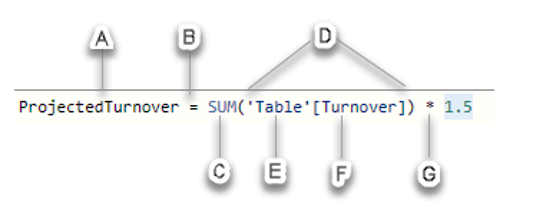automation

# DAX Coding – Behind the scenes of Power BIRavi Kumar, our Product Application Manager, provides an insight into how Power BI is brought to life.

3 mins

Now that we’ve introduced you to the Power Platform suite, let’s take a deeper look into the coding that brings Power BI to life.

What is DAX coding?

Data Analysis Expressions (DAX) is a functional language designed by Microsoft which is used in Power BI and other data visualisation tools. As its name suggests, DAX is essentially expressions or formulas that are used for data analysis and calculations. These expressions are a collection and combination of functions, operators and constants that are evaluated as one formula to yield results.

DAX coding functions

Functions within DAX are predefined formulas that perform calculations on values provided to it. There are over two hundred functions that can be used for doing different things and they can be as simple as linking together two text values using the concatenate function [CONCATENATE (<text 1>, <text 2>)]. There is an extensive list of DAX functions such as 'CALENDAR', 'COUNT', 'AVERAGE', 'FORMAT', 'VALUES' and many more.

Projected Turnover

Not too dissimilar to Excel formulas, most of DAX is written after an equal sign (=). The formulas have two primary data types: numeric (integers, decimals and currency) and non-numeric. However, they can take other forms such as strings and binary objects. The below image depicts an example of a DAX formula, the purpose of which is to create a column [ProjectedTurnover] in a table to store the calculated value.

A - Name of the measure.

B – Operating sign shows the start of the DAX formula.

C - It is the DAX function used to add the values of a given field (Turnover) from a table (Table). The function used here is SUM.

D - The parenthesis () is used to enclose and define arguments in an expression. Every function must have at least one argument.

E - It is the name of the table from which a field or column is taken in the formula (Table).

F - It is the name of the field from which the formula will use the values. For instance, the function SUM will apply itself on the values of the column or field [Turnover] of the table Sales.

G - It is another operator used for multiplication. Although, the syntax elements from A to F forms the basic syntax of DAX.

DAX formulas and Power BI

DAX formulas are extremely useful for tools like Power BI as they help data analysts maximise the value of their data by visualising trends, providing actionable insights and speeding up the decision-making process. This then allows you to solve your business problems efficiently. In terms of utilising DAX formulas in Power BI, there are three main ways it can be used: calculated tables, calculated columns and measures.

Calculated tables will add an additional table to a report, calculated columns will add a column to a table, and measures add a summary or aggregated measure to a table. The main difference between these three calculation types is their context and the outputs they generate.

There is a lot more around DAX coding that can be explored, however, this blog has hopefully given you a brief insight into what goes on behind the scenes of Power BI.